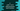# C program to print from 1 to N using recursive main function### C program to print from 1 to N using recursive main :

In this tutorial, we will learn how to print 1 to N using recursive main method.Recursive main means, our program will call the main() method recursively. Let’s take a look at the program :

### C program :

``````#include <stdio.h>

int main()
{
//1
static int no = 1;
static int max = -1;

//2
if (max == -1)
{
printf("Enter value of N : ");
scanf("%d", &max);
}

//3
if (no <= max)
{
printf("%d ", no++);
main();
}
}``````

### Explanation :

The commented numbers in the above program denote the step number below :

1. Create one static number no to store the value of current number and one static integer max to store the maximum number the program will print. We are declaring these integers as static because these are initialized for only once.It remain until the end of the program.
2. If the maximum value is not set or if max = -1, ask the user to enter the value of maximum value and store it in variable max.
3. Check if the current no is less than or equal to the maximum value max, print the value of current no. Also, increment the value of no by 1. After that call the main() again recursively. It will run recursively till all numbers are printed.

### Sample Output :

``````Enter value of N : 12
1 2 3 4 5 6 7 8 9 10 11 12

Enter value of N : 10
1 2 3 4 5 6 7 8 9 10
``````